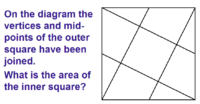# Area of inner square problem

#### apple2357

##### Full MemberCan anyone help with this problem? I have found an approach and got an answer which i have checked and it is correct but i wonder if there are other approaches? My method involved just geometric arguments but i am not fully convinced i can conclude what 'looks' to be the case as fact. I will explain what i mean with a spoiler below. But please ignore before you have looked at it for yourself please.

SPOILER DOWN BELOW...
.
.
.
.
.
.
.
.
.
SPOILER...

Looking at the picture it appears that a trapezium and a triangle can be rearranged to make the same as the inner square. This means that there are 5 inner squares. So the inner square is a 1/5 of the whole bigger square.

#### lev888

##### Full Member
I came up with the same solution. Which part is not clear?

#### apple2357

##### Full Member
I came up with the same solution. Which part is not clear?
How can i be sure that a trapezium and triangle make the same as the inner square?

#### lev888

##### Full Member
How can i be sure that a trapezium and triangle make the same as the inner square?
1. Prove that the combined shapes are squares and have equal sizes
2. Prove that the inner square has the same size (obvious)

#### apple2357

##### Full Member
Ok, so that's where i am stuck i guess! Can i use something on similar/congruent shapes and midpoints?

#### Dr.Peterson

##### Elite Member
There are many ways you might do it; one is just to draw another copy of the entire figure adjacent to it on the right, and observe how parts fit together. Another would be to calculate the lengths of various segments and use them to find area of each piece.

#### ksdhart2

##### Senior Member
I solved it in a completely different way altogether. I started by drawing the figure to scale on graph paper with the dimensions of the square fixed to be 1x1. This, then, results in the vertices of the inner square being at (0.2, 0.4); (0.4, 0.8); (0.8, 0.6); and (0.6, 0.2). The area of this square is 0.2, so I conclude that the area of the inner square must be 20% of the area of the whole square.

#### lev888

##### Full Member
Square - a plane figure with four equal straight sides and four right angles.
Take one combined shape. Can you prove that all angles are right and sides are straight and equal?

#### MarkFL

##### Super Moderator
Staff member
We can see from one of the four smaller right triangles inside the outer square, that the side length $$s$$ of the inner square may be found from:

$$\displaystyle \left(\frac{s}{2}\right)^2+s^2=\left(\frac{S}{2}\right)^2$$

Note: $$S$$ is the side length of the outer square.

$$\displaystyle 5s^2=S^2$$

$$\displaystyle s^2=\frac{1}{5}S^2$$

And so yes, we find that the inner square has 1/5 the area of the outer square.

•Subhotosh Khan

#### apple2357

##### Full Member
We can see from one of the four smaller right triangles inside the outer square, that the side length $$s$$ of the inner square may be found from:

$$\displaystyle \left(\frac{s}{2}\right)^2+s^2=\left(\frac{S}{2}\right)^2$$

Note: $$S$$ is the side length of the outer square.

$$\displaystyle 5s^2=S^2$$

$$\displaystyle s^2=\frac{1}{5}S^2$$

And so yes, we find that the inner square has 1/5 the area of the outer square.

Where does the (s/2) come from in the first line? How do we know this? Sorry i am being dopey!

#### lev888

##### Full Member
Where does the (s/2) come from in the first line? How do we know this? Sorry i am being dopey!
S is the side of the large square. S/2 is the hypotenuse of the small triangle. Do you know why?
Small s is the side of the inner square. If we prove that the larger leg of the small triangle is also s, then the smaller leg is s/2. Do you know why?
Last step is just the Pythagorean theorem applied to the small triangle.

#### apple2357

##### Full Member
Ok, This is the key bit for me:

If we prove that the larger leg of the small triangle is also s

I think i can see this by identifying congruent triangles by constructing a new triangle under the inner square with has a hypotenuse of s ?

I may be overthinking it!

#### lev888

##### Full Member
I added a triangle. It's easy to prove that it's congruent to the small triangles. Its larger leg is equal to the side of the inner square.

#### Attachments

• 1.6 MB Views: 1

#### apple2357

##### Full Member
Ah yes! Thank you!

#### JeffM

##### Elite Member
•topsquark

#### LCKurtz

##### Junior Member
This property holds for any parallelogram, not just a square. The inner one is 1/5 the area of the larger one. There is a complete thread on this problem on Physics Forums at:

•apple2357

#### lev888

##### Full Member
Labels? We don't need no stinkin' labels!

•JeffM